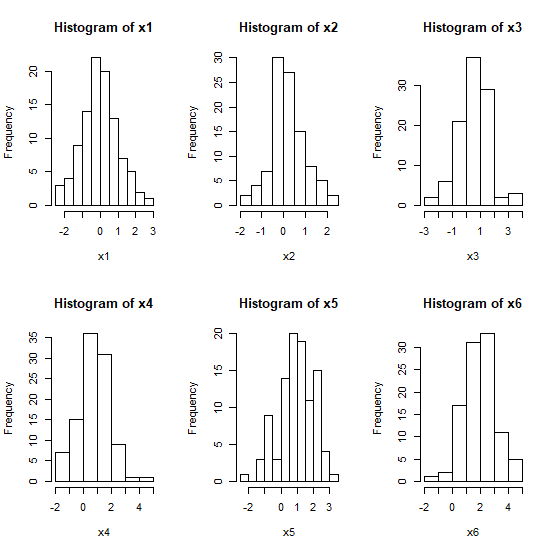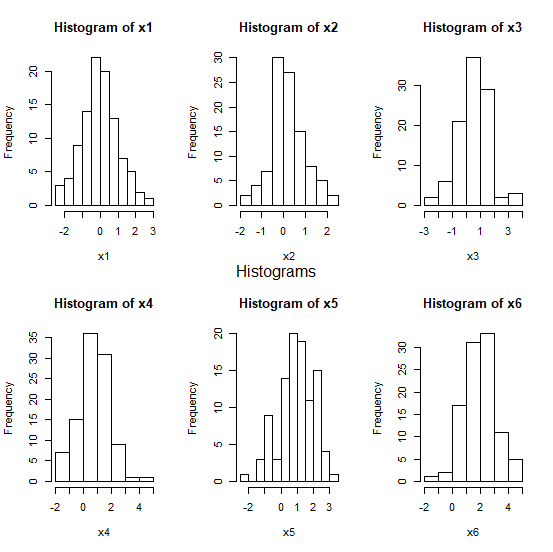# How to write a common title for par(mfrow) plots in R?

We can create multiple plots using par(mfrow) on a single plot window in R. It might be possible that all of these plots are different or same as well. Irrespective of the type of plots, we can give a common title to all the plots. This can be a situation where we want to show, say, scatterplots, and histograms of few variables but the object of our analysis is fixed. To write a common title between plots in the plot window we can use mtext and adjust the title position by changing line argument.

## Example

set.seed(100)
x1<-rnorm(100)
x2<-rnorm(100,0.2)
x3<-rnorm(100,0.5)
x4<-rnorm(100,0.8)
x5<-rnorm(100,1.1)
x6<-rnorm(100,1.8)
par(mfrow=c(2,3))
hist(x1)
hist(x2)
hist(x3)
hist(x4)
hist(x5)
hist(x6)

## OutputThe code to write a common title “Histograms” between the top and bottom histograms −

## Example

mtext("Histograms",side=3,line=-22,outer=TRUE)

## OutputUpdated on: 21-Aug-2020

1K+ Views